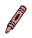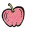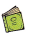All the solutions provided in McGraw Hill Math Grade 1 Answer Key PDF Chapter 2 Lesson 6 Addition Word Problems as per the latest syllabus guidelines.

Solve

Add to solve each problem. Use the number line to help.

Question 1.
Sam has 7.
Ana has 2.
How manyin all?
7 + 2 = 9Question 2.
There are 4in a bowl. There are 2. in a bag. How manyin all?
4 + 2 = ____Given that
There are 4in a bowl. There are 2. in a bag.
4 + 2 = 6
Therefore there are 6 apples in all.

Question 3.
Dan haš 6. Lily has 8. How manyare there?
6 + 8 = _____Dan haš 6. Lily has 8.